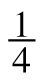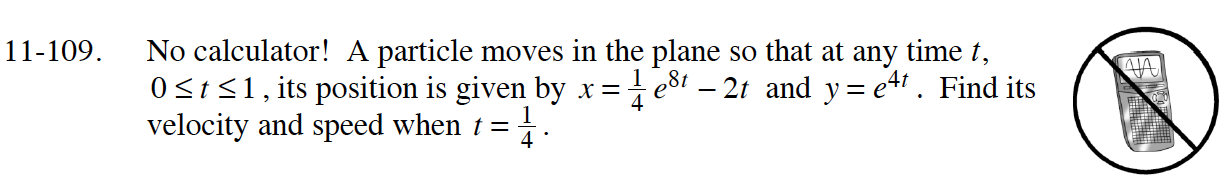### Home > CALC > Chapter 11 > Lesson 11.3.2 > Problem11-109

11-109.

No calculator! A particle moves in the plane so that at any time t, 0 ≤ t ≤ 1, its position is givenx =e 8t − 2t and y = e 4t . Find its velocity and speed when t =. Homework Help ✎$\text{speed}=\sqrt{(x^\prime(t))^2+(y^\prime(t))^2}$